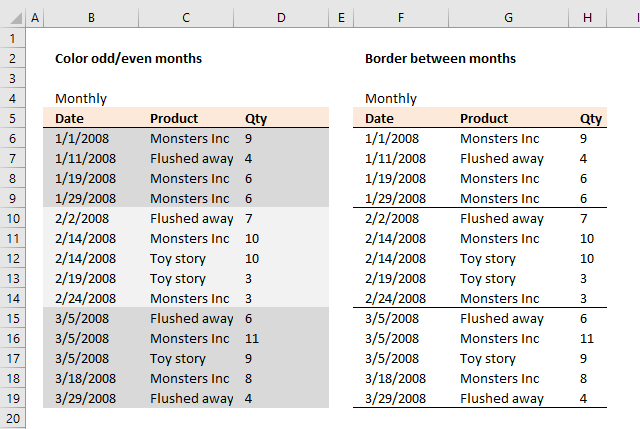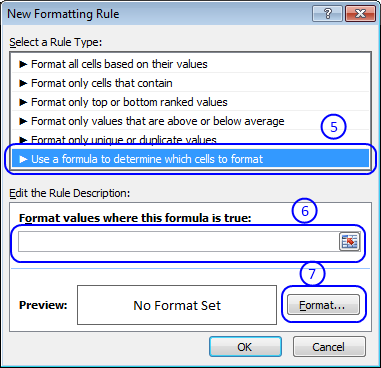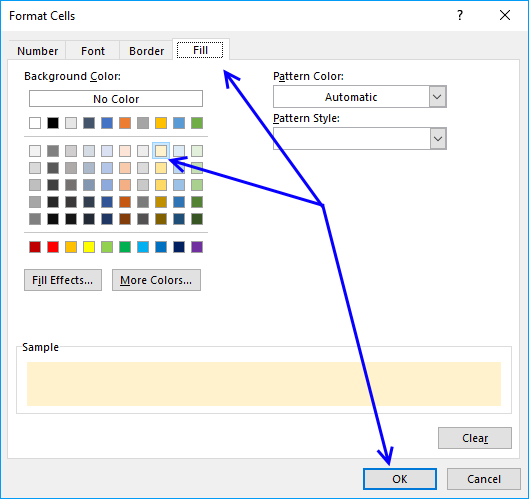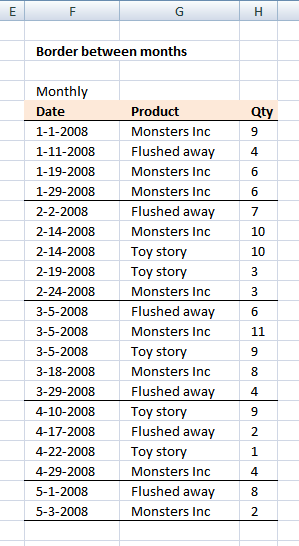Author: Oscar Cronquist Article last updated on December 10, 2018### Color odd months

Conditional formatting formula:

=MOD(MONTH(\$B6),2)

### Explaining CF formula in cell B6

#### Step 1 - Calculate number of month

The MONTH function returns a number representing a month based on a date. 1 = January, 2 = February ... 12 = December.

MONTH(\$B6)

becomes

MONTH(1/1/2008)

becomes

MONTH(39448)

and returns 1. 1 = January.

#### Step 2 - Calculate remainder

The MOD function returns the remainder after a number is divided by a divisor.

MOD(MONTH(\$B6),2)

becomes

MOD(1, 2)

and returns 1.

In cell B10 the date changes to 2/2/2008. MOD(MONTH(\$B6),2) becomes MOD(2,2) and returns 0 (zero). This cell is not highlighted with this specific color.

### How to apply conditional formatting

1. Select cell range B6:D19
2. Go to tab "Home" on the ribbon
3. Press with mouse on "Conditional Formatting" button
4. Press with mouse on "New Rule.."
5. Select "Use a formula to determine which cells to format"6. Type =MOD(MONTH(\$B6),2) in field "Format values where this formula is true:"
7. Then press with left mouse button on "Format..." button8. Go to tab "Fill"
9. Pick a color
10. Press with left mouse button on OK button
11. Press with left mouse button on OK button to return to Excel.

### Color even months

Conditional formatting formula:

=NOT(MOD(MONTH(\$B6),2))

The NOT function returns the boolean opposite to the given argument. The numerical equivalent to boolean value TRUE is 1 and FALSE is 0 (zero).### Color odd years

Conditional formatting formula:

=MOD(YEAR(\$B6),2)

The YEAR function returns the year based on a date.

### Color even years

Conditional formatting formula:

=NOT(MOD(YEAR(\$B6),2))### Border between months

Conditional formatting formula:

=MONTH(\$F7)<>MONTH(\$F6)### Border between years

Conditional formatting formula:

=YEAR(\$F33)<>YEAR(\$F32)

### Get excel file for this tutorial.

Highlight every even month.xlsx
(Excel 2007 Workbook *.xlsx)